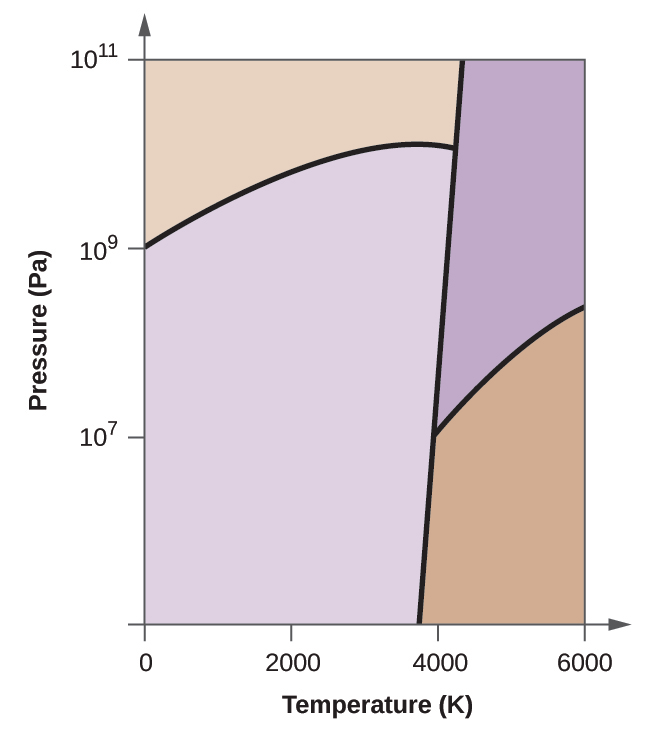# Problem: Elemental carbon has one gas phase, one liquid phase, and two different solid phases, as shown in the phase diagram:(f) If the temperature of a sample of carbon increases from 3000 K to 5000 K at a constant pressure of 106 Pa, which phase transition occurs, if any?

###### FREE Expert Solution
93% (124 ratings)
###### Problem Details

Elemental carbon has one gas phase, one liquid phase, and two different solid phases, as shown in the phase diagram:(f) If the temperature of a sample of carbon increases from 3000 K to 5000 K at a constant pressure of 106 Pa, which phase transition occurs, if any?

Frequently Asked Questions

What scientific concept do you need to know in order to solve this problem?

Our tutors have indicated that to solve this problem you will need to apply the Phase Diagram concept. You can view video lessons to learn Phase Diagram. Or if you need more Phase Diagram practice, you can also practice Phase Diagram practice problems.

What textbook is this problem found in?

Our data indicates that this problem or a close variation was asked in Chemistry - OpenStax 2015th Edition. You can also practice Chemistry - OpenStax 2015th Edition practice problems.